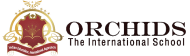Enquire Now

# Discount Calculator

#### Formula

Discount = Cost price – Selling Price
Cost Price(c.p.) :
Sellig Price(s.p.) :
Discount(c.p. - s.p.) :
Discount % :

Discount Calculator is a free online calculator tool by which you can find the percentage of discounts with the help of cost price and selling price. It makes your calculation easy and accurate. It also displays the result in a fraction of a second. When you go shopping and see any dress whose price was 1900, you get it in just 1200. Do you know how much discount you are getting? It may take time to calculate it manually, but the Discount calculator makes your task hassle-free. To find the real deal, you get the discount result in seconds using this calculator.

What is Discount?
A discount is when a certain amount or percentage is taken off of an item's regular price. When you go shopping during a festival, they will give you some amount of reduction on the original price. That is called a discount.
THE FORMULA FOR DISCOUNT
Discount = Cost price – Selling Price
Example of Discount
Suppose you are at the supermarket, and you want to buy cookies. The cost of cookies is 650, but you get them for just 420 rs. Then what is the discount you got?
Given values are
• Cost Price = 650
• Selling Price = 420
• Discount = ?
• Let’s put values on formula
• Discount = Cost price – Selling Price
• Discount = 650 - 420
• Discount = 230
• Discount Percentage = 35 %
How to Use Discount Calculator?
1. Enter the value of the cost price.
2. Enter the value of the selling price.
3. Click on the “calculate” button.

## FAQs

##### What is the selling price?

| K12 Techno Services ®

ORCHIDS - The International School | Terms | Privacy Policy | Cancellation